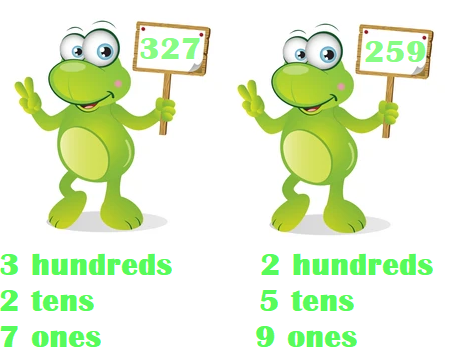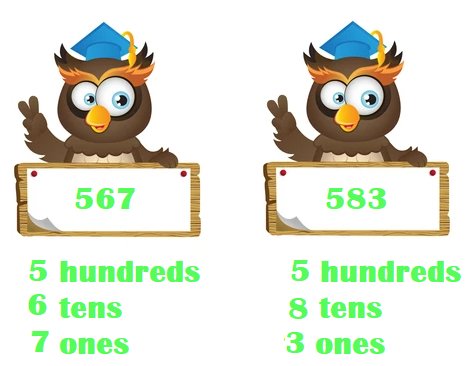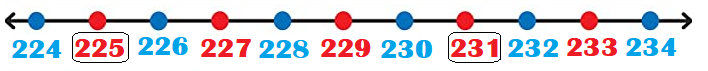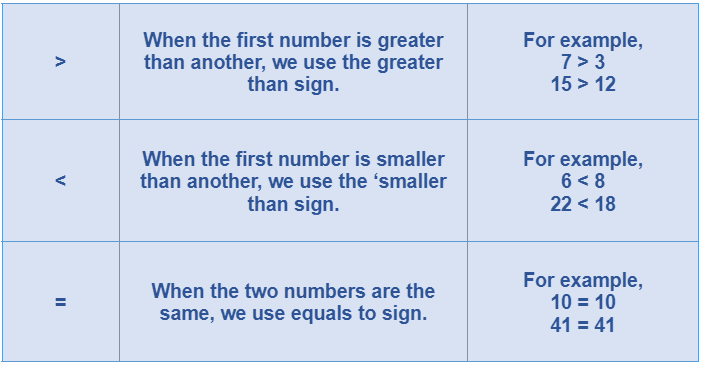# Math > Year 3

## Comparing numbers up to 1,000

What is Comparing?

To compare means to know if one number is the same as, or smaller than, or bigger than, another number.

How do we compare numbers?
To compare the number, we can partition the numbers.

For example,We start comparing numbers from the highest value column i.e., from the left-hand side.

327 has 3 hundreds and 259 has 2 hundreds.

So, 327 is bigger than 259.

What if the hundreds are the same?
If the hundreds are the same, we’ll compare the next value column i.e., ‘tens’.567 and 583 have 5 hundreds each. So, we’ll look at the tens digit now.
567 has ‘6 tens’ and 583 has ‘8 tens’.
So, 567 is smaller than 583.

Comparing numbers using a number line

We can also use number lines to compare two numbers.

For example,The number on the right side of a number line is always bigger than the number on the left side.
So, 231 is bigger than 225.

SymbolsThe easiest way to remember these signs is to remember that the open side of the sign ‘>’ and ‘<’ points toward a bigger number and the closed side of the sign ‘>’ and ‘<’ points towards a smaller number.

Let's try a question.

Given below is the distance of 3 towns from Cambridge. The closest town is

a. Town A = 547km
b. Town B = 517 km
c. Town C = 528 km
d. Town D = 514km

Explanation:

The closest town means the one with the smallest distance.
All the distances have 5 at hundreds place. So, we’ll look at the tens column.
Town A has 4 tens.
Town B has 1 ten.
Town C has 2 tens.
Town D has 1 tens.
This eliminates Town A and Town C.
Let us compare the one’s column of Town B and D.
Town B has 7 tens and Town D has 4 ones.
Hence, Town D is closest to the town.

//